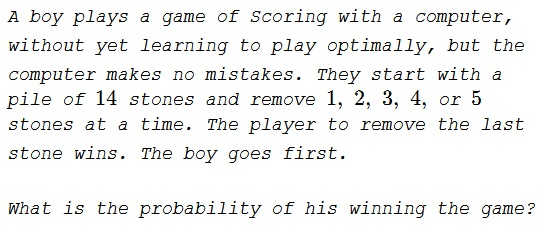# Probability in Scoring

### Problem### Solution 1

The game of Scoring is a great tool for learning the modulo arithmetic. It's best analyzed starting from the end. The last move was the removal of $1,$ $2,$ $3,$ $4,$ or $5$ stones. Thus the previous player has left less than $6$ stones. If he left $6$ he would win. If he left more than $6,$ the other player would be able to leave $6$ stones and win.

So whoever manages to leave $6$ stones wins the game. Put another way, whoever takes the seventh stone wins. We can now apply the previous argument but replacing "first" with "seventh" and continue backwards. The right strategy is then to leave a multiple of $6$ after each move. If you do not, the other player will.

The game starts with $14$ stones, the boy should take $2.$ If he does not he loses right away.

If he does, whichever move computer does next, the boy has one in six chance to make the only right move. The same applies to the next two moves computer/boy.

However they play, the right strategy is to leave a multiple of $6.$ On the first move the boy does that with the probability of $\displaystyle \frac{1}{5}$ (he needs to remove $2$ and leave $12$ stones.) From there on there remain two pairs of moves, meaning the that boy yet has two opportunities to make a wrong move. As before, there's a one in five chance to make a right move. Thus the boy does that with the probability of $\displaystyle \frac{1}{5}\cdot\frac{1}{5}\cdot\frac{1}{5}=\frac{1}{125}.$

We may observe that the boy may reach the state of six stones with the probability of $\displaystyle \frac{1}{5^2}$ (hitting $2$ on the first move and whatever it takes to get to $6,$ depending on computer's move). After computer's move (probably random) the boy may be left with $1,$ $2,$ $3,$ $4,$ or $5$ stones. Assuming that even the fellow who is yet to master the necessary skills to play Scoring, is still clever enough to remove all the remaining stones in order to win the game, at this point he wins it with certainty, making the final probability of the win equal to $\displaystyle \frac{1}{5^2}.$

### Solution 2

If a player "A" gets the stone count to $6$, that player optimally forces a win. Player "B" can only leave $1-5$, and in the final move, player "A" picks all the stones.

With this observation, we note that if in the first move, the boy gets the stone count to $13$, $11$, $10$ and $9$, the computer can force a win. For in the cases $9-11$, the computer can bring the stone count down to $6$ in a single move. For the case of $13$, the computer can bring the stone count to $12$ making $7-11$ possible moves for the boy. The computer can then force a $6$ irrespective of the stone count that the boy leaves.

Thus, we focus on the case where the boy picks $2$ stones in the first step and gets the count down to $12$. This first move has probability $P_1=1/5$. In this case, the boy always has an optimal path (not necessarily the chosen path) to victory. No matter what the computer chooses, the boy has only $1$ in $5$ ways of getting to $6$. In all other cases, the boy either has to leave a count greater than $6$ with $6$ accessible to the opponent in a single move, or a count less than $6$ such that all the stones can be picked up in one move. Thus, the probability of the boy winning in this case if $P_2=1/5$.

Hence, the probability of the boy winning is $P_1\cdot P_2=1/25$.

### Acknowledgment

This is a paraphrase of problem 65264 from a Russian problem collection. David Koops observed that on the last move the boy has yet to make a right move and that is not obvious that he will.

Solution 2 is by Amit Itagi.